The topic of Forces is usually taught in Primary 6 and I realised that many P6 students struggle to answer questions when two or more forces are involved.

As such, in today’s article, I will introduce the template answer required to tackle such questions.

## What Do You Need To Know

Students are required to have an understanding on the four main types of forces:

• Gravitational Force
• Frictional Force/Friction
• Magnetic Force and
• Elastic Spring Force

Most students are competent in answering questions that involve only one type of force.

However, most examination questions do not simply test individual forces acting independently on the object. Questions typically test students on how two or more forces acting on the object interact. For these questions, students are usually able to identify which of the four types of forces are at work but encounter difficulties when they are structuring their answers.

## Key Words/ Concepts

When there are 2 or more forces at work, there are a few steps students can follow:

Step 1: Identify what type of forces are at work.

Step 2: Identify the direction of the forces (Left? Right? Up? Down?)

Step 3: Group the forces together according to their direction.

Step 4: Identify which group of forces is stronger.

Step 5: Apply the template answer:

“The ________ force acting on the ________ (object) is greater than the ________ force acting on the ________ (object).”

That’s it!

With that, students will be able to nail similar questions using this step-by-step guide.

## Let’s Take A Look At This Question Below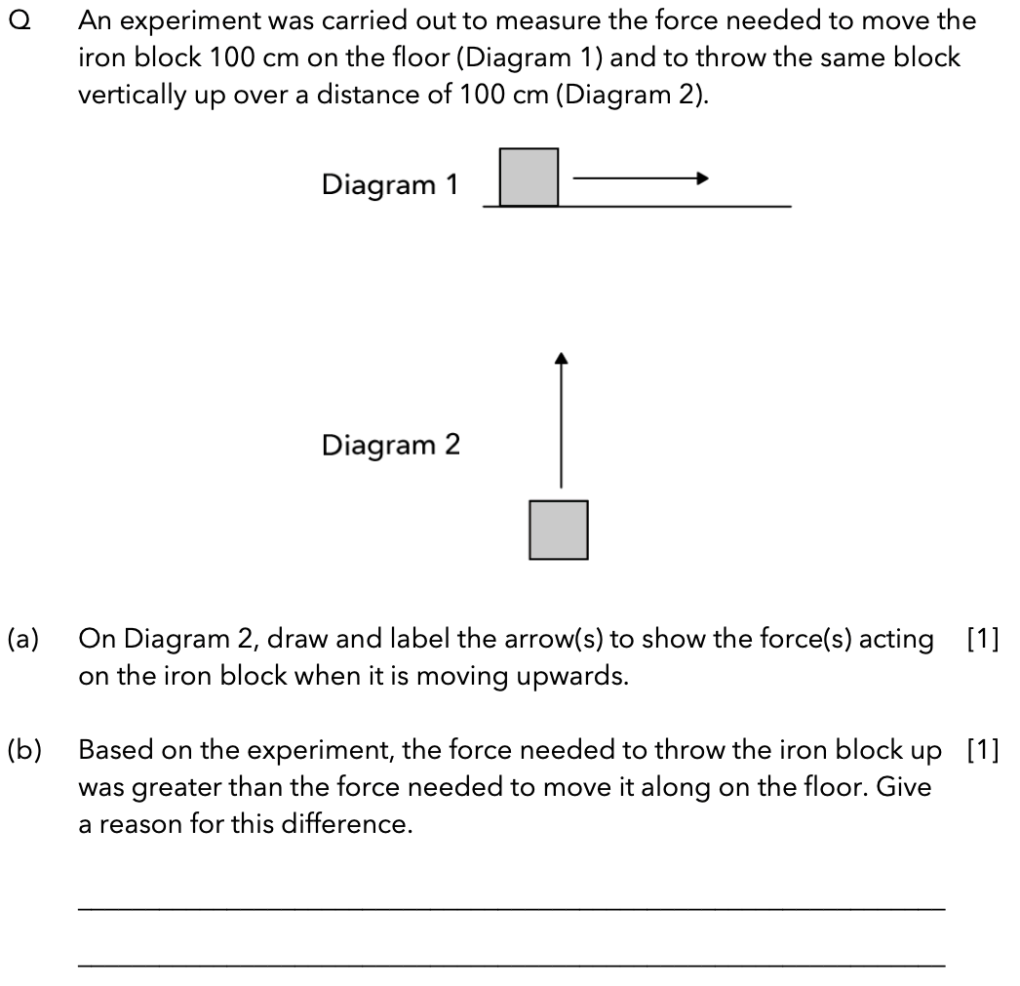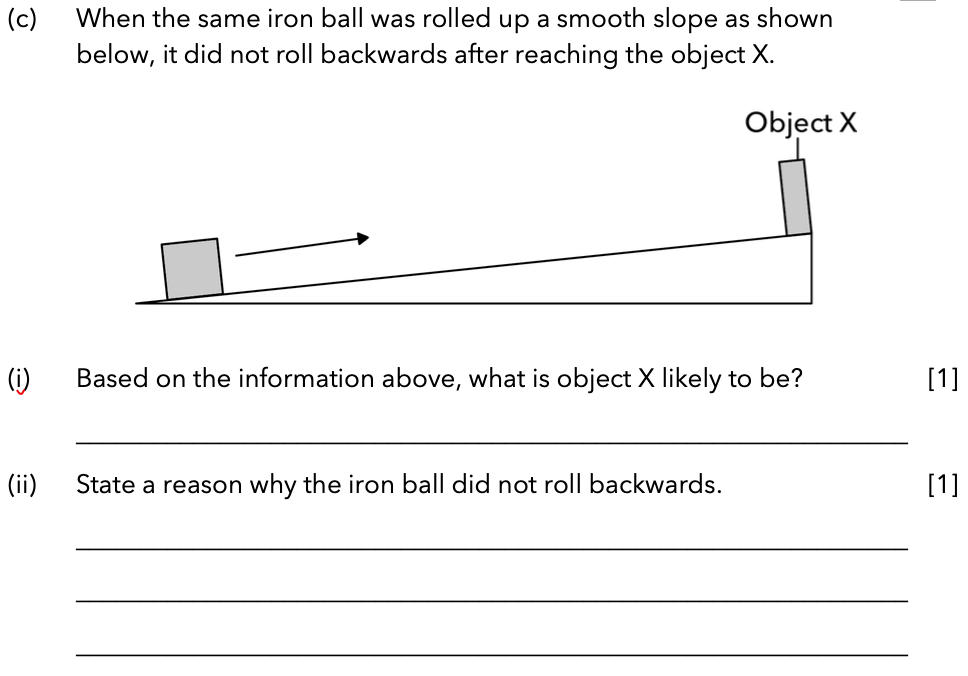Source: Nan Hua Primary School – 2015 P6 SA2 Science Examination Paper [Q40] (ADAPTED)

### Part (A)

Question: On Diagram 2, draw and label the arrow(s) to show the force(s) acting on the iron block when it is moving upwards.

Besides the push force acting on the iron block, there are two other forces acting on the block.

• Gravitational force (acts on all objects at all times)
• Gravitational force is a downwards pulling force that acts on objects.
• Air resistance (a form of frictional force)
• Friction opposes motion. As the block is moving upwards, air resistance is acting downwards on the iron block.### Part (B)

Question: Based on the experiment, the force needed to throw the iron block up was greater than the force needed to move it along on the floor. Give a reason for this difference.

The forces acting on the iron block in both diagrams are summarised in the table below: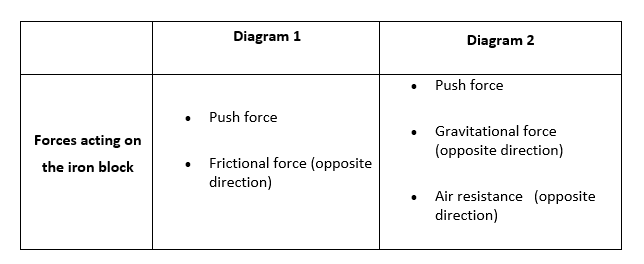In Diagram 1, for the iron block to move along the floor, the push force acting on the block needs to be greater than the frictional force between the surface of the block and the floor.

• Push force acting on the block only needs to be greater than 1 force acting in the opposite direction

In Diagram 2, for the iron block to move upwards in the air, the push force acting on the block needs to be greater than both the gravitational force and the air resistance acting on the block.

• Push force acting on the block needs to be greater than 2 forces acting in the opposite direction

Hence the answer to part (b) would be:

When the block was thrown upwards, the push force acting on the block needed to be greater than both gravitational force and air resistance acting on the block. On the other hand, the push force acting on the block that is moving along the floor only needs to be greater than frictional force between the surface of the block and the ground.

### Part (C – Part One)

Question: When the same iron block was pushed up a smooth slope as shown below, it did not move backwards after reaching object X. Based on the information above, what is object X likely to be?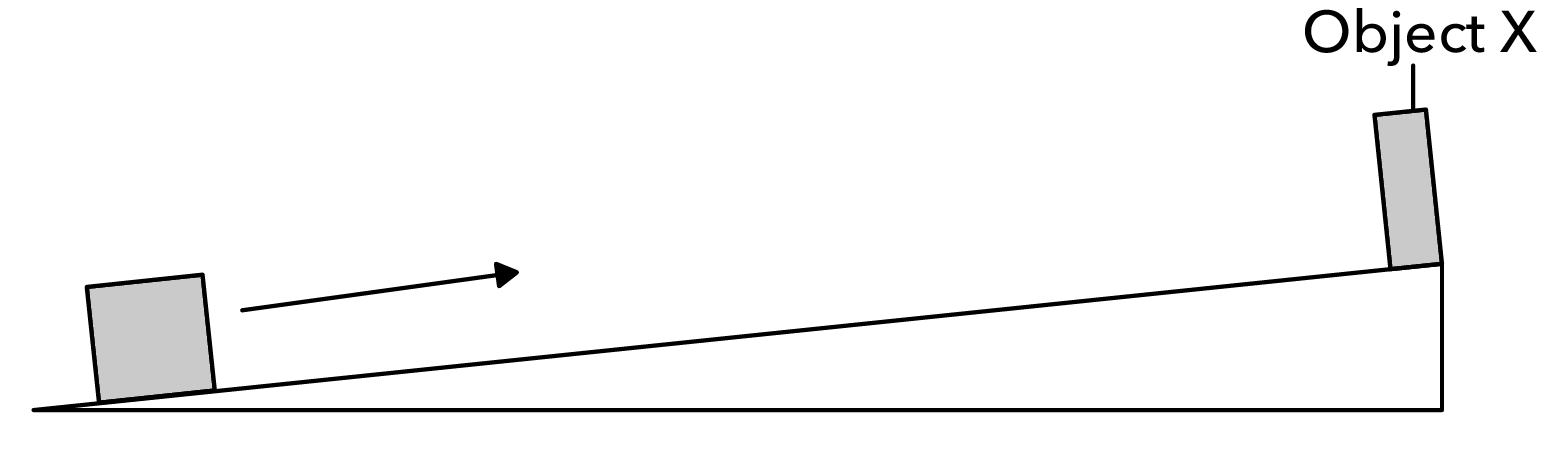Since the iron block is a magnetic material, object X must be a magnet, which caused the iron block to remain attracted to it and not move downwards.

Hence the answer to part (ci) would be:

A magnet.”

### Part (C – Part Two)

Question: State a reason why the iron block did not move backwards.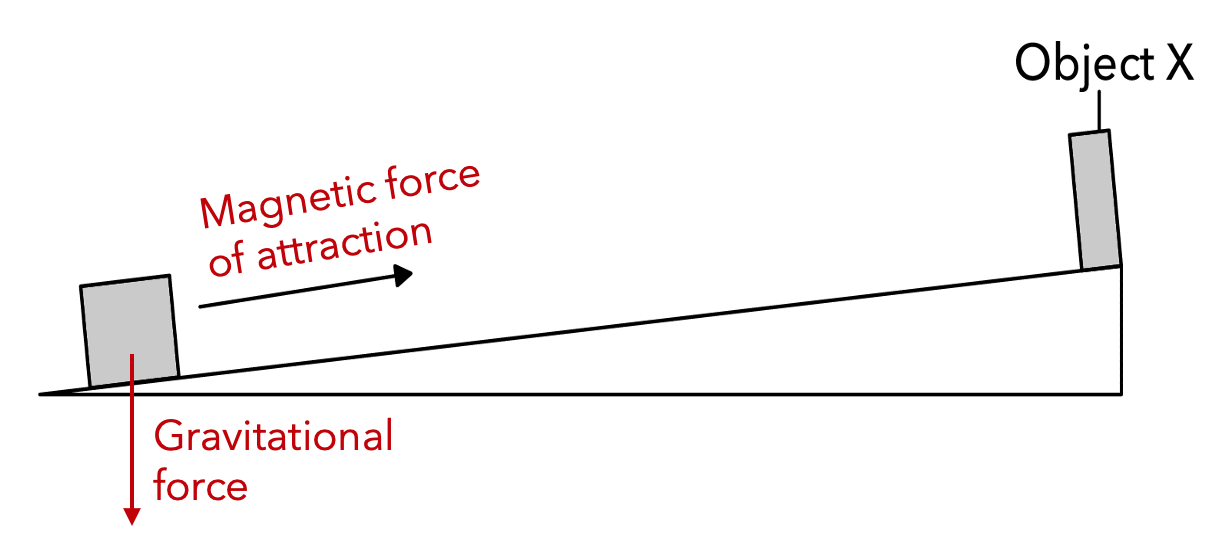There are two forces acting on the iron block.

• Gravitational force (acts on all objects at all times)
• Gravitational force is a downwards pulling force that acts on objects.
• Magnetic force of attraction
• An upwards pulling force which kept the block from moving downwards.

Since the two forces are acting in the opposite direction and the iron block did not move backwards, the stronger force has to be the magnetic force of attraction.

Hence, the answer to Part (cii) will be:

The magnetic force of attraction acting on the iron block was greater than the gravitational force acting on it, preventing it from moving backwards.

I hope that this article has taught you how to tackle questions on Forces using this simple 5-step guide!If you like our methodology, we’ve some upcoming workshops: